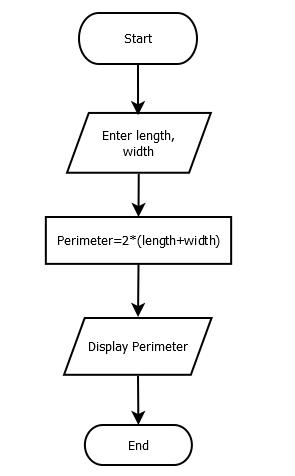# Write a Program to Find perimeter of Rectangle

Perimeter of a rectangle can be found by multiplying 2 by sum of its length and width. You can refer Algorithm and flowchart of this program as well for better understanding.

#### Flowchart:#### Algorithm:

Begin:

Step1: Take two numeric values (length and width) from the user

Step2: Multiply by 2 to sum of both values and store result into a new variable

Step3: Display the result

End:

#### Program Example

This program is tested and executed using Atom IDE with Python 3.8 version. To find perimeter, we cast user input to float because input function returns string type value.

l=float(input('Enter length of rectangle'))
b=float(input('Enter width of rectangle'))
perimeter = 2*(l+b)
print("Perimeter = ",perimeter)
Output:
Enter length of rectangle 10
Enter width of rectangle 20
Perimeter = 60.0
Trending